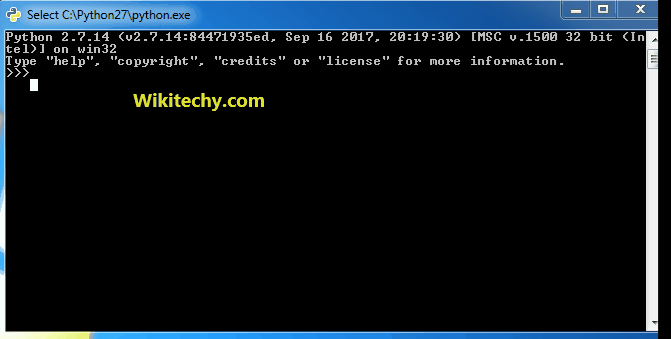# python tutorial - Iterator Python | Iterator Functions in Python islice(), starmap(), tee() - learn python - python programming

1. islice(iterable, start, stop, step) :- This iterator selectively prints the values mentioned in its iterable container passed as argument. This iterator takes 4 arguments, iterable container, starting pos., ending position and step.

2. starmap(func., tuple list) :- This iterator takes a function and tuple list as argument and returns the value according to the function from each tuple of list.Learn Python - Python tutorial - python iterator tools - Python examples - Python programs

python - Sample - python code :

python tutorial - Output :

```The sliced list values are : [4, 7, 10]
The values acc. to function are : [1, 1, 4, 1]
```

3. takewhile(func, iterable) :- This iterator is opposite of dropwhile(), it prints the values till the function returns false for 1st time.

4. tee(iterator, count) :- This iterator splits the container into a number of iterators mentioned in the argument.

python - Sample - python code :

python tutorial - Output :

```The list values till 1st false value are : [2, 4, 6]
The iterators are :
[2, 4, 6, 7, 8, 10, 20]
[2, 4, 6, 7, 8, 10, 20]
[2, 4, 6, 7, 8, 10, 20]
```

5. zip_longest( iterable1, iterable2, fillval.) :- This iterator prints the values of iterables alternatively in sequence. If one of the iterables is printed fully, remaining values are filled by the values assigned to fillvalue.

python - Sample - python code :

python tutorial - Output :

```The combined values of iterables is  :
('W', 'i') ('k', 'i') ('t', 'e') ('c', 'h') ('y', '_')
```

Combinatoric Iterators

1. product(iter1, iter2) :- This iterator prints the cartesian product of the two iterable containers passed as arguments.

2. permutations(iter, group_size) :- This iterator prints all possible permutation of all elements of iterable. The size of each permuted group is decided by group_size argument.

python - Sample - python code :

python tutorial - Output :

```The cartesian product of the containers is :
[('A', '1'), ('A', '2'), ('B', '1'), ('B', '2')]
All the permutations of the given container is :
[('W', 'T'), ('W', 'Y'), ('T', 'W'), ('T', 'Y'), ('Y', 'W'), ('Y', 'T')]
```

3. combinations(iterable, group_size) :- This iterator prints all the possible combinations(without replacement) of the container passed in arguments in the specified group size in sorted order.

4. combinations_with_replacement(iterable, group_size) :- This iterator prints all the possible combinations(with replacement) of the container passed in arguments in the specified group size in sorted order.

python - Sample - python code :

python tutorial - Output :

```All the combination of container in sorted order(without replacement) is :
[('1', '2'), ('1', '3'), ('1', '4'), ('2', '3'), ('2', '4'), ('3', '4')]
All the combination of container in sorted order(with replacement) is :
[('W', 'W'), ('W', 'T'), ('W', 'Y'), ('T', 'T'), ('T', 'Y'), ('Y', 'Y')]
```

Infinite Iterators

1. count(start, step) :- This iterator starts printing from the “start” number and prints infinitely. If steps are mentioned, the numbers are skipped else step is 1 by default.

Example :

```iterator.count(5,2) prints -- 5,7,9,11...infinitely
```

2. cycle(iterable) :- This iterator prints all values in order from the passed container. It restarts printing from beginning again when all elements are printed in a cyclic manner.

Example :

```iterator.cycle([1,2,3,4]) prints -- 1,2,3,4,1,2,3,4,1...infinitely
```

3. repeat(val, num) :- This iterator repeatedly prints the passed value infinite number of times. If num. is mentioned, them till that number.

python - Sample - python code :

python tutorial - Output :

```Printing the numbers repeatedly :
[25, 25, 25, 25]
```

Wikitechy tutorial site provides you all the learn python , python wiki , python loop , python with , python switch , print python , python import , python os , learning python book , python book online , python programming training course , python programming course online , online python training course , python training online